## How to Calculate and Solve for Rated Static Head (for Gating at Mould Parting) | Design of Gating System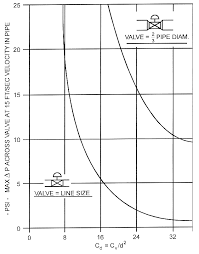The image above represents rated static head (for Gating at Mould Parting).

To compute for rated static head, two essential parameters are needed and these parameters are Height of the Sprue from its Top to the Point of Metal Entry into the Mould Cavity (H) and Height of the Casting (C).

The formula for calculating rated static head (for gating at mould parting).

Hs = H – C8

Where:

Hs = Rated Static Head (for gating at mould parting)
H = Height of the Sprue from its Top to the Point of Metal Entry into the Mould Cavity
C = Height of Casting

Let’s solve an example;
Find the rated static head (for gating at mould parting) when the height of the sprue from its top to the point of metal entry into the mould cavity is 11 and the height of casting is 19.

This implies that;

H = Height of the Sprue from its Top to the Point of Metal Entry into the Mould Cavity = 11
C = Height of Casting = 19

Hs = H – C8
Hs = 11 – 19 / 8
Hs = 11 – 2.375
Hs = 8.625

Therefore, the rated static head (for gating at mould parting) is 8.625 m.

Calculating the Height of the Sprue from its Top to the Point of Metal Entry into the Mould Cavity when the Rated Static Head (for gating at mould parting) and the Height of Casting is Given.

H = Hs + C8

Where;

H = Height of the Sprue from its Top to the Point of Metal Entry into the Mould Cavity
Hs = Rated Static Head (for gating at mould parting)
C = Height of Casting

Let’s solve an example;
Find the height of the sprue from its top to the point of metal entry into the mould cavity when the rated static head is 22 and the height of casting is 8.

This implies that;

Hs = Rated Static Head (for gating at mould parting) = 22
C = Height of Casting = 8

H = Hs + C8
H = 22 + 88
H = 22 + 1
H = 23

Therefore, the height of the sprue from its top to the point of metal entry into the mould cavity is 23 m.

Calculating the Height of Casting when the Rated Static Head (for gating at mould parting) and the Height of the Sprue from its Top to the Point of Metal Entry into the Mould Cavity is Given.

c = – (Hs – H) 8

Where;

C = Height of Casting
Hs = Rated Static Head (for gating at mould parting)
H = Height of the Sprue from its Top to the Point of Metal Entry into the Mould Cavity

Let’s solve an example;
Find the height of casting when the rated static head is 40 and the height of the sprue from its top of the point of metal entry into the mould cavity is 24.

This implies that;

Hs = Rated Static Head (for gating at mould parting) = 40
H = Height of the Sprue from its Top to the Point of Metal Entry into the Mould Cavity = 24

c = – (Hs – H) 8
c = – (40 – 24) 8
c = – (16) 8
c = – 128

Therefore, the height of casting is – 128 m.

Nickzom Calculator – The Calculator Encyclopedia is capable of calculating the rated static head (for gating at mould parting).

To get the answer and workings of the rated static head (for gating at mould parting) using the Nickzom Calculator – The Calculator Encyclopedia. First, you need to obtain the app.

You can get this app via any of these means:

To get access to the professional version via web, you need to register and subscribe for NGN 1,500 per annum to have utter access to all functionalities.
You can also try the demo version via https://www.nickzom.org/calculator

Apple (Paid) – https://itunes.apple.com/us/app/nickzom-calculator/id1331162702?mt=8
Once, you have obtained the calculator encyclopedia app, proceed to the Calculator Map, then click on Materials & Metallurgical under Engineering.Now, Click on Foundry Technology under Material & MetallurgicalNow, Click on Design of Gating System under Foundry TechnologyNow, Click on Rated Static Head (for gating at mould parting) under Design of Gating SystemThe screenshot below displays the page or activity to enter your values, to get the answer for the rated static head (for gating at mould parting) according to the respective parameters which are the Height of the Sprue from its Top to the Point of Metal Entry into the Mould Cavity (H) and Height of the Casting (C).Now, enter the values appropriately and accordingly for the parameters as required by the Height of the Sprue from its Top to the Point of Metal Entry into the Mould Cavity (H) is 11 and Height of the Casting (C) is 19.Finally, Click on Calculate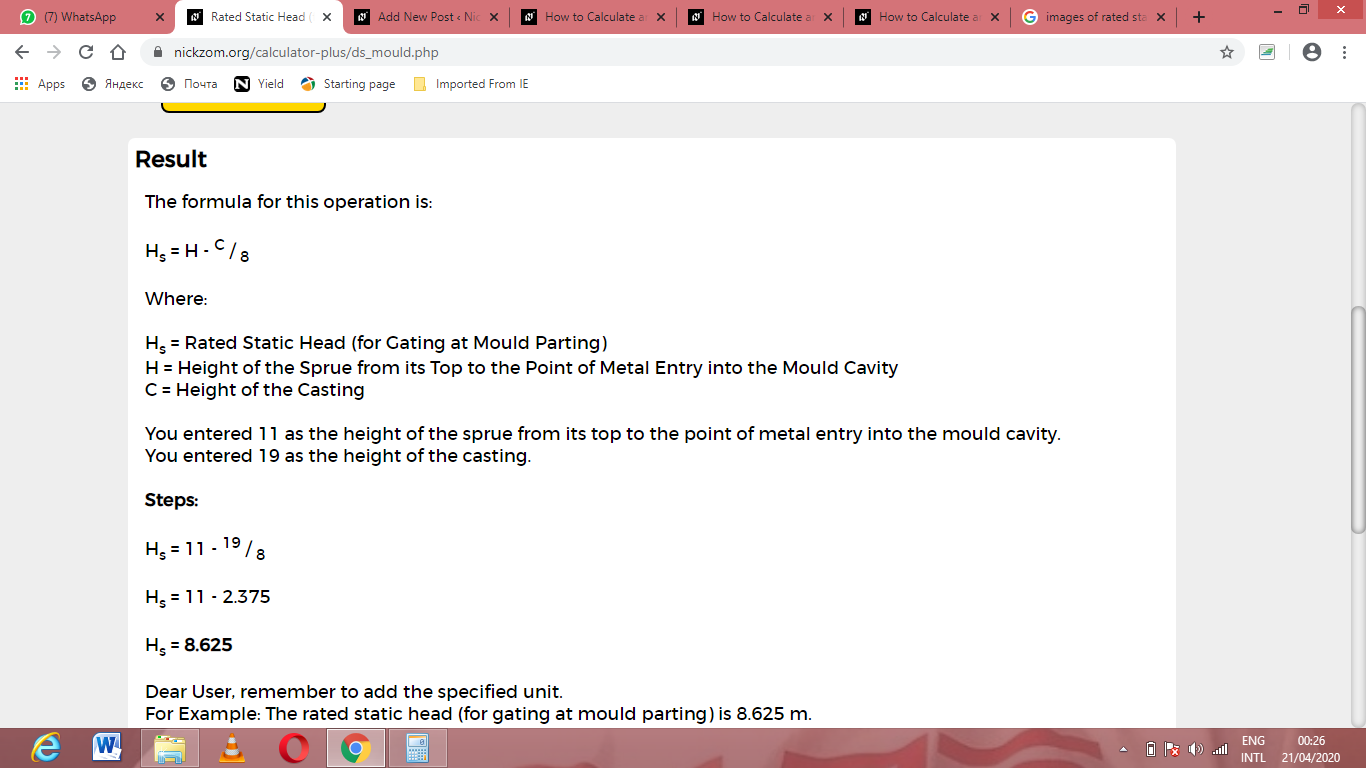As you can see from the screenshot above, Nickzom Calculator– The Calculator Encyclopedia solves for the rated static head (for gating at mould parting) and presents the formula, workings and steps too.

## How to Calculate and Solve for Rated Static Head (in Top Gating) | Design of Gating System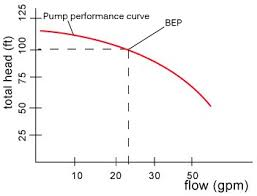The image represents rated static head (in top gating).

To compute for rated static head (int top gating), one essential parameter is needed and the parameter is Height of the Sprue from its Top to the Point of Metal Entry into the Mould Cavity (H).

The formula for calculating rated static head (in top gating).

Hs = H

Where:

Hs = Rated Static Head (in top Gating)
H = Height of the Sprue from its Top to the Point of Metal Entry into the Mould Cavity

Let’s solve an example;
Find the rated static head (in top gating) when the height of the sprue from its top to the point of metal entry into the mould cavity is 10.

This implies that;

H = Height of the Sprue from its Top to the Point of Metal Entry into the Mould Cavity = 10

Hs = H
Hs = 10

Therefore, the rated static head (in top gating) is 10.

## How to Calculate and Solve for Rated Static Head (in Bottom Gating) | Design of Gating System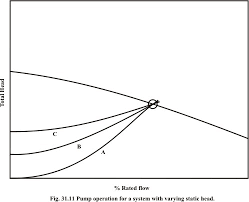The image above represents rated static head (in bottom gating).

To compute for rated static head, two essential parameters are needed and these parameters are Height of the Sprue from its Top to the Point of Metal Entry into the Mould Cavity (H) and Height of the Casting (C).

The formula for calculating rated static head (in bottom gating).

Hs = H – C / 2

Where:

Hs = Rated Static Head (in Bottom Gating)
H = Height of the Sprue from its Top to the Point of Metal Entry into the Mould Cavity
C = Height of Casting

Let’s solve an example;
Find the rated static head (in bottom gating) when the height of the sprue from its top to the point of metal entry into the mould cavity is 11 and the height of casting is 8.

This implies that;

H = Height of the Sprue from its Top to the Point of Metal Entry into the Mould Cavity = 11
C = Height of Casting = 8

Hs = H – C / 2
Hs = 11 – 8 / 2
Hs = 11 – 4
Hs = 7

Therefore, the rated static head (in bottom gating) is 7 m.

Calculating the Height of the Sprue from its Top to the Point of Metal Entry into the Mould Cavity when the Rated Static Head (in bottom gating) and the Height of Casting is Given.

H = HsC / 2

Where;

H = Height of the Sprue from its Top to the Point of Metal Entry into the Mould Cavity
Hs = Rated Static Head (in Bottom Gating)
C = Height of Casting

Let’s solve an example;
Find the height of the sprue from its top to the point of metal entry into the mould cavity when the rated static head is 22 and the height of casting is 8.

This implies that;

Hs = Rated Static Head (in Bottom Gating) = 22
C = Height of Casting = 8

H = HsC / 2
H = 22 + 8 / 2
H = 22 + 4
H = 26

Therefore, the height of the sprue from its top to the point of metal entry into the mould cavity is 26 m.

## How to Calculate and Solve for Rated Static Head | Design of Gating System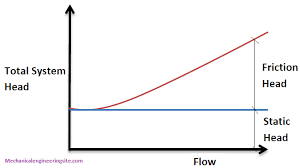The image above represents rated static head.

To compute for rated static head, three essential parameters are needed and these parameters are Height of the Sprue from its Top to the Point of Metal Entry into the Mould Cavity (H), Height of the Casting System from its top (P) and Height of the Casting (C).

The formula for calculating rated static head:

Hs = H – P2 / 2C

Where:

H = Height of the Sprue from its Top to the Point of Metal Entry into the Mould Cavity
P = Height of the Casting from its Top
C = Height of the Casting

Let’s solve an example;
Find the rated static head when the height of the sprue from its top to the point of metal entry into the mould cavity is 9,the height of the casting from its top is 5 and the height of the casting is 11.

This implies that;

H = Height of the Sprue from its Top to the Point of Metal Entry into the Mould Cavity = 9
P = Height of the Casting from its Top = 5
C = Height of the Casting = 11

Hs = H – P2 / 2C
Hs = 9 – 52 / 2 x 11
Hs = 9 – 25 / 22
Hs = 9 – 1.136
Hs = 7.86

Therefore, the rated static head is 7.86 m.

Calculating the Height of the Sprue from its Top to the Point of Metal Entry into the Mould Cavity when the Rated Static Head, Height of the Casting System from its Top and the Height of the Casting is Given.

H = HsP2 / 2C

Where;

H = Height of the Sprue from its Top to the Point of Metal Entry into the Mould Cavity
P = Height of the Casting from its Top
C = Height of the Casting

Let’s solve an example;
Find the height of the sprue when the rated static head is 25, the height of the casting system from its top is 8 and the height of the casting is 4.

This implies that;

Hs = Rated Static Head = 25
P = Height of the Casting from its Top = 8
C = Height of the Casting = 4

H = HsP2 / 2C
H = 25 + 82 / 2 x 4
H = 25 + 64 / 8
H = 25 + 8
H = 33

Therefore, the height of the casting system from its top is 33.

## How to Calculate and Solve for Total Cross-Sectional Areas of Gates | Design of Gating System by a Hydraulic Method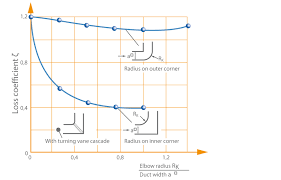The image above represents total cross-sectional areas of gates.

To compute for total cross-sectional areas of gates, four essential parameters are needed and these parameters are mass of the casting (G), density of melt (ρ), pouring time (t) and pouring speed (v).

The formula for calculating total cross-sectional areas of gates:

Fg = G / ρtv

Where:

Fg = Total Cross-Sectional Area of Gates
G = Mass of the Casting
ρ = Density of Melt
t = Pouring Time
v = Pouring Speed

Let’s solve an example;
Find the total cross-sectional areas of gates when mass of the casting is 12, density of melt is 16, pouring time is 5 and pouring speed is 25.

This implies that;

G = Mass of the Casting = 12
ρ = Density of Melt = 16
t = Pouring Time = 5
v = Pouring Speed = 25

Fg = G / ρtv
Fg = 12 / 16 x 5 x 25
Fg = 12 / 2000
Fg = 0.006

Therefore, the total cross-sectional areas of gates is 0.006 m2.

Calculating the Mass of the Casting when the Total Cross-Sectional Areas of Gates, the Density of Melt, the Pouring Time and the Pouring Speed is Given.

G = Fg x ρtv

Where;

G = Mass of the Casting
Fg = Total Cross-Sectional Area of Gates
ρ = Density of Melt
t = Pouring Time
v = Pouring Speed

Let’s solve an example;
Find the mass of the casting when the total cross-sectional areas of gates is 32, the density of melt is 4, the pouring time is 10 and the pouring speed is 2.

This implies that;

Fg = Total Cross-Sectional Area of Gates = 32
ρ = Density of Melt = 4
t = Pouring Time  = 10
v = Pouring Speed = 2

G = Fg x ρtv
G = 32 x 4 x 10 x 2
G = 2560

Therefore, the mass of the Casting is 2560 m.

## How to Calculate and Solve for Flexural Strength for Rectangular Cross-section in Defects | Ceramics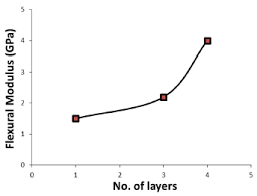The image above represents flexural strength for rectangular cross-section in defects.

To compute for flexural strength for rectangular cross-section in defect, four essential parameters are needed and these parameters are Load at fracture (Ff), Length of the cross-section (b), Width of the cross-section (d) and Distance between support points (L).

The formula for calculating flexural strength for rectangular cross-section in defects:

σfs = 3FfL / 2bd²

Where:

σfs = Flexural Strength
L = Distance between Support Points
b = Length of Cross-section
d = Width of Cross-section

Let’s solve an example;
Find the flexural strength when the load at fracture is 10, the length of cross-section is 7, width of cross-section is 9 and the distance between support points is 21.

This implies that;

Ff = Load at Fracture = 10
L = Distance between Support Points = 21
b = Length of Cross-section = 7
d = Width of Cross-section = 9

σfs = 3FfL / 2bd²
σfr = 3(10)(21) / 2(7)(9)²
σfr = (630) / 2(7)(81)
σfr = (630) / (1134)
σfr = 0.55

Therefore, the flexural strength for rectangular cross-section in defect is 0.55 Pa.

Calculating the Distance Between Support Points when the Flexural Strength for Rectangular Cross-Section in Defects, Load at Fracture, length of the cross-section and width of cross-section is Given.

L = σfs x 2bd2 / 3Ff

Where;

L = Distance between Support Points
σfs = Flexural Strength
b = Length of Cross-section
d = Width of Cross-section

Let’s solve an example;
Find the distance between support points when the flexural strength is 20, the load at fracture is 12, the length of cross-section is 7 and the width of cross-section is 9.

This implies that;

σfs = Flexural Strength = 20
Ff = Load at Fracture = 12
b = Length of Cross-section = 7
d = Width of Cross-section = 9

L = σfs x 2bd2 / 3Ff
L = 20 x 2 x 7 x 92 / 3 x 12
L = 280 x 81 / 36
L = 22680 / 36
L = 630

Therefore, the distance between support points is 630.

## How to Calculate and Solve for Flexural Strength for Circular Cross-section in Defects | Ceramics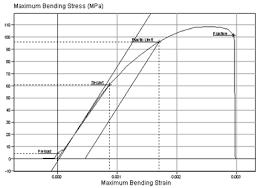The image above represents flexural strength for circular cross-section in defects.

To compute for flexural strength for circular cross-section in defects, three essential parameters are needed and these parameters are Load at fracture (Ff), Specimen radius (R) and Distance between support Points (L).

The formula for calculating flexural strength for circular cross-section in defects:

σfs = FfL / πR³

Where:

σfs = Flexural Strength
L = Distance between Support Points

Lets’s solve an example;
Find the flexural strength when the distance between support points is 30, load at fracture is 21 and the specimen radius is 11.

This implies that;

L = Distance between Support Points = 30
Ff = Load at Fracture = 21
R = Specimen Radius = 11

σfs = FfL / πR³
σfs = (21)(30) / π(11)³
σfs = (630) / π(1331)
σfs = (630) / (4181.4)
σfs = 0.150

Therefore, the flexural strength for circular cross-section is 0.150 Pa.

Calculating the Distance Between Support Points when the Flexural Strength for Circular Cross-section, the Specimen Radius and the Load at Fracture is Given.

L = σfs x πR³ / Ff

Where;

L = Distance between Support Points
σfs = Flexural Strength

Let’s solve an example;
Find the distance between support points when the flexural strength is 44, load at fracture is 3 and specimen radius is 20.

This implies that;

σfs = Flexural Strength = 44
Ff = Load at Fracture = 3
R = Specimen Radius = 20

L = σfs x πR³ / Ff
L = 44 x π x 3³ / 20
L = 44 x π x 27 / 20
L = 3732.2 / 20
L = 186.61

Therefore, the distance between support point is 186.61.

## How to Calculate and Solve for Flexural Strength with Relation to Volume Fraction | Ceramics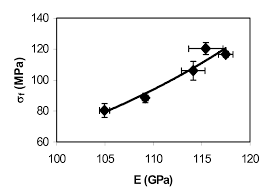The image above represents Flexural strength with relation to volume.

To compute for flexural strength with relation to volume, three essential parameters are needed and these parameters are Initial Stress (σo), Constant (n) and Volume fraction porosity (P).

The formula for calculating flexural strength with relation to volume:

σfs = σo exp (-nP)

Where:

σfs = Flexural Strength
σo = Initial Stress
n = Constant
P = Volume Fraction Porosity

Let’s solve an example;
Find the flexural strength when the initial stress is 11, the constant is 8 and the volume fraction porosity is 22.

This implies that;

σo = Initial Stress = 11
n = Constant = 8
P = Volume Fraction Porosity = 22

σfs = σo exp (-nP)
σfr = (11)exp(-(8)(22))
σfr = (11)exp(-176)
σfr = (11)(3.665e-77)
σfr = 4.032e-76

Therefore, the flexural strength is 4.032e-76 Pa.

Calculating the Initial Stress when the Flexural Strength, the Constant and the Volume Fraction Porosity is Given.

σo = σfr / exp (-nP)

Where;

σo = Initial Stress
σfs = Flexural Strength
n = Constant
P = Volume Fraction Porosity

Let’s solve an example;
Find the initial stress when the flexural strength is 20, the constant is 10 and the volume fraction porosity is 8.

This implies that;

σfs = Flexural Strength = 20
n = Constant = 10
P = Volume Fraction Porosity = 8

σo = σfr / exp (-nP)
σo = 20 / exp (-10 x 8)
σo = 20 / exp (-80)
σo = 20 / – 5.54e+34
σo = – 3.61e-34

Therefore, the initial stress is 3.61e-34.

## How to Calculate and Solve for Relationship between Modulus of Elasticity on Volume Fraction Porosity, E | Ceramics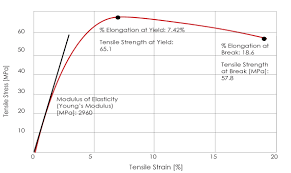The image above represents modulus of elasticity.

To compute for the relationship between modulus of elasticity on volume fraction porosity, two essential parameters are needed and these are Modulus of Elasticity of Non Porous Material (Eo) and Volume Fraction Porosity (P).

The formula for calculating the modulus of elasticity:

E = Eo(1 – 1.9P + 0.9P²)

Where:

E = Modulus of Elasticity
Eo = Modulus of Elasticity of Non Porous Material.
P = Volume Fraction Porosity

Let’s solve an example;
Find the modulus of elasticity when the modulus of elasticity of non porous material is 12 and volume fraction porosity is 22.

This implies that;

Eo = Modulus of Elasticity of Non Porous Material = 12
P = Volume Fraction Porosity = 22

E = Eo(1 – 1.9P + 0.9P²)
E = (12)(1 – 1.9(22) + 0.9(22)²)
E = (12)(1 – (41.8) + 0.9(484))
E = (12)(1 – (41.8) + (435.6))
E = (12)(394.8)
E = 4737.6

Therefore, the modulus of elasticity is 4737.6 Pa.

## How to Calculate and Solve for Viscosity | Ceramics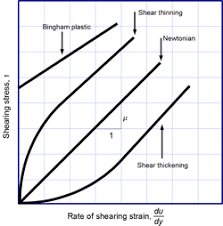The image above represents viscosity.

To compute for viscosity, three essential parameters are needed and these parameters are Force applied (F), Area (A) and Derivation Ratio of Velocity to Distance of Fluid Flow (dv/dy).

The formula for calculating viscosity:

η = F/A / dv/dy

Where:

η = Viscosity
F = Force Applied
A = Area
dv/dy = Derivation Ratio of Velocity to Distance of Fluid Flow

Let’s solve an example;
Find the viscosity when the force applied is 21, area is 14 and derivation ratio of velocity to distance of fluid flow is 19.

This implies that;

F = Force Applied = 21
A = Area = 14
dv/dy = Derivation Ratio of Velocity to Distance of Fluid Flow = 19

η = F/A / dv/dy
η = (21/14) / (19)
η = (1.5) / (19)
η = 0.0789

Therefore, the viscosity is 0.0789 Pa s.

Calculating Force Applied when the Viscosity, the Area and the Derivation ratio of velocity to distance of fluid flow is Given.

F = (η x dv/dy) A

Where;

F = Force Applied
η = Viscosity
A = Area
dv/dy = Derivation Ratio of Velocity to Distance of Fluid Flow

Let’s solve an example;
Find the force applied when the viscosity is 20, the area is 30 and the derivation is 8.

This implies that;

η = Viscosity = 20
A = Area = 30
dv/dy = Derivation Ratio of Velocity to Distance of Fluid Flow = 8

F = (η x dv/dy) A
F = (20 x 8) 30
F = (160) 30
F = 4800

Therefore, the force applied is 4800.